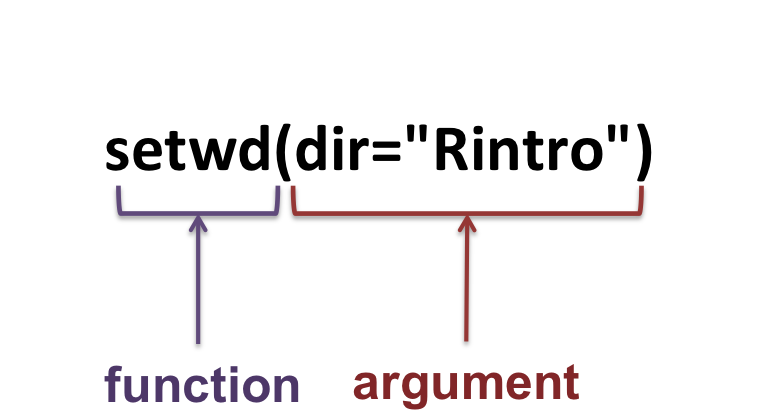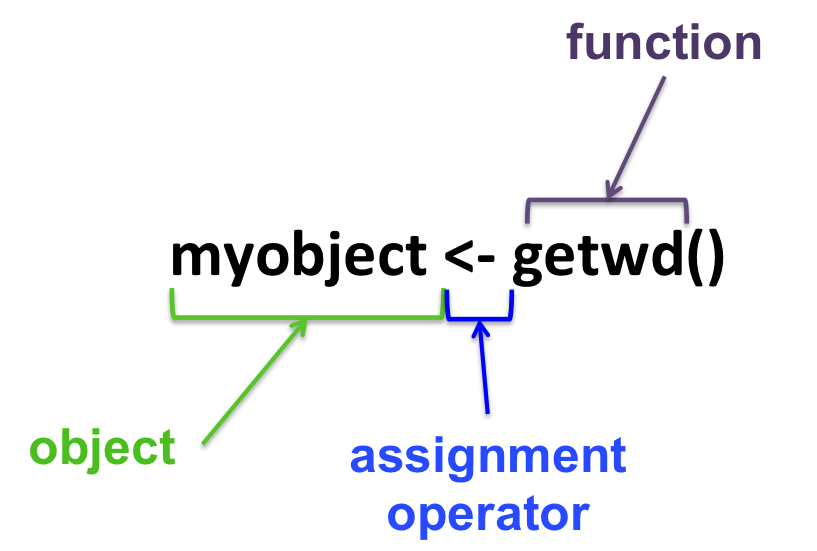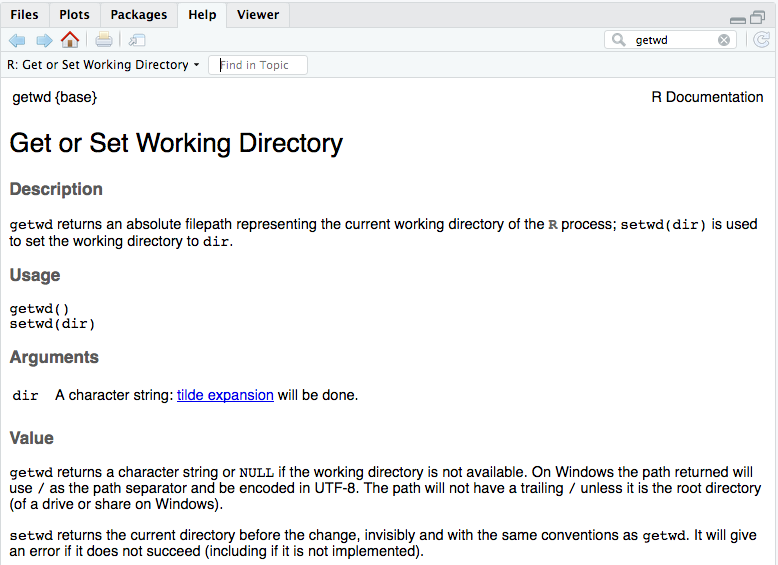# Part6 Functions

In programming, a function is a section of a program that performs a specific task.

For example, the function getwd is used as:

``getwd()``

and has the task of outputting the current working directory.

You can recognize a function with the round brackets: function()

A function can also take arguments/parameters

``setwd(dir="Rcourse")``

setwd changes the current working directory and takes one argument dir.• Assign the output of a function to an object:• Getting help:

From the console:

``````help(getwd)
?getwd``````

From the RStudio bottom-right panel:• The help pages show:
• required/optional argument(s), if any.
• default values for each argument(s), if any.
• examples.
• detailed description.
• Get the example of a function:
``example(mean)``

• Note on arguments

The help page shows the compulsory arguments in the Usage section: in the help page of getwd and setwd (above), you can see that getwd doesn’t take any compulsory argument, and setwd takes one compulsory argument that is called dir.

Compulsory arguments can be given with their names: in such case you don’t need to respect a specific order, or without their names, in which case you have to respect the order specified in the help page!

For example, the rep.int function (a variant of the rep function) takes 2 arguments (see in help page): x and times, in that order:

``````# use arguments with their names:
rep.int(x=1, times=3)``````
``##  1 1 1``
``````# use arguments with their names without respecting the order:
rep.int(times=3, x=1)``````
``##  1 1 1``
``````# use arguments without their names but respecting the order:
rep.int(1, 3)``````
``##  1 1 1``
``````# use arguments without their names without respecting the order:
rep.int(3, 1)``````
``##  3``
``# It works, but is not giving the expected output!``